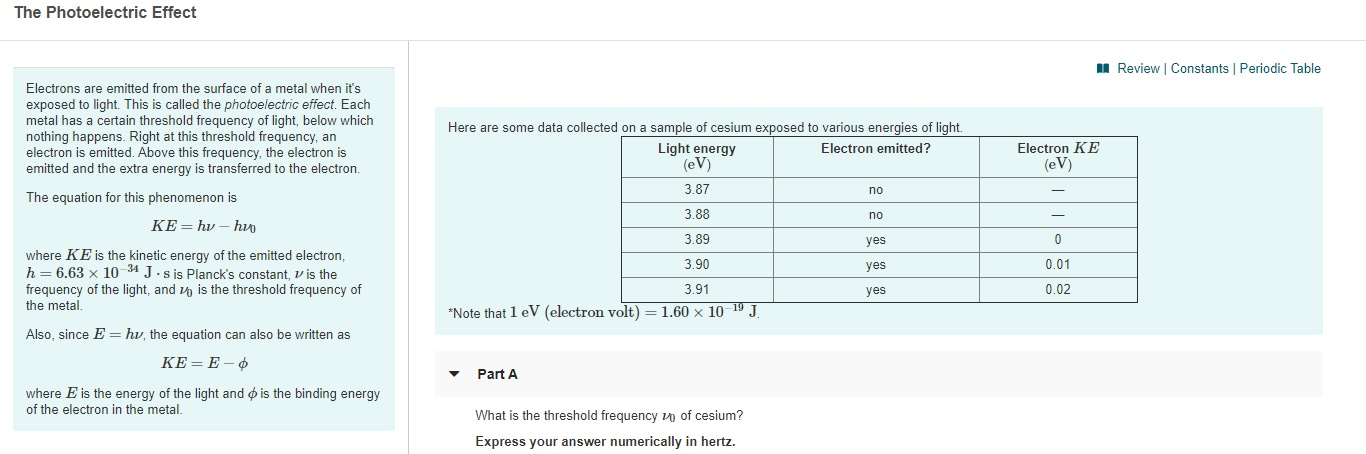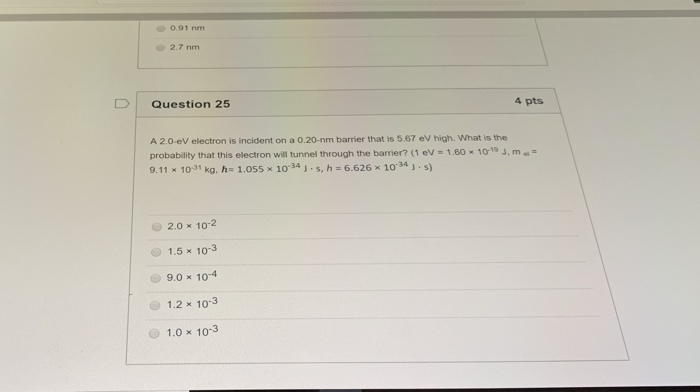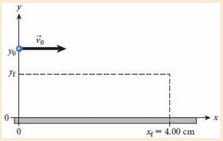# What is the threshold frequency ν0 of cesium? Note that 1 eV (electron volt)=1.60×10−19 J. Express...

What is the threshold frequency ν0 of cesium? Note that 1 eV (electron volt)=1.60×10−19 J. Express your answer numerically in hertz.

Concepts and reason

Calculate the threshold frequency by using the following equation.

${\rm{KE = h}}\nu {\rm{ + h}}{\nu _{\rm{o}}}$

For threshold energy, kinetic energy will be zero.

$\begin{array}{c}\\{\rm{h}}\nu {\rm{ = h}}{\nu _{\rm{o}}}\\\\{\rm{E = h}}{{\rm{\nu }}_{\rm{o}}}\\\end{array}$

Here, h is plank constant, ${\nu _{\rm{o}}}$ is threshold frequency, and E is ionization energy.

Calculate the threshold frequency by using the values of plank constant and the energy.

Fundamentals

Minimum amount of frequency that causes photo electric emission is called as threshold frequency.

Emission of electrons when light is incident on surface of a material is called as photo emission and this effect is called as photo electric effect.

Ionization energy of cesium is ${\rm{375}}{\rm{.7 kJ/mol}}$ .

$\begin{array}{c}\\{\rm{Energy per atom = }}375.7{\rm{ }}\frac{{{\rm{kJ}}}}{{{\rm{mol}}}}{\rm{ }} \times \left( {\frac{{{\rm{1}}{{\rm{0}}^{\rm{3}}}{\rm{ J}}}}{{{\rm{1 kJ}}}}} \right) \times \left( {\frac{{{\rm{1 mol}}}}{{{\rm{6}}{\rm{.023 \times 1}}{{\rm{0}}^{{\rm{23}}}}{\rm{ atoms}}}}} \right)\\\\ = {\rm{ }}6.24 \times {10^{ - 19}}{\rm{ J/atom}}\\\end{array}$

Substitute the values in the formula.

$\begin{array}{c}\\6.24 \times {10^{ - 19}}{\rm{ J/atom}} = \left( {{\rm{6}}{\rm{.626 \times 1}}{{\rm{0}}^{ - {\rm{34}}}}{\rm{ }}{{\rm{m}}^{\rm{2}}}{\rm{kg/s}}} \right) \times {\nu _{\rm{o}}}\\\\6.24 \times {10^{ - 19}}{\rm{ J}} \times \frac{{{\rm{kg}}{\rm{.}}{{\rm{m}}^2}/{{\rm{s}}^2}}}{{1{\rm{J}}}} = \left( {{\rm{6}}{\rm{.626 \times 1}}{{\rm{0}}^{ - {\rm{34}}}}{\rm{ }}{{\rm{m}}^{\rm{2}}}{\rm{kg/s}}} \right) \times {\nu _{\rm{o}}}\\\\{\nu _{\rm{o}}} = \frac{{6.24 \times {{10}^{ - 19}}{\rm{kg}}{\rm{.}}{{\rm{m}}^2}/{{\rm{s}}^2}}}{{{\rm{6}}{\rm{.626 \times 1}}{{\rm{0}}^{ - {\rm{34}}}}{\rm{ }}{{\rm{m}}^{\rm{2}}}{\rm{kg/s}}}}\\\\{\nu _{\rm{o}}} = 9.42 \times {10^{14}}{{\rm{s}}^{ - 1}}\\\end{array}$

One cycle per second is equal to hertz. So, the threshold frequency of cesium is $9.42 \times {10^{14}}{\rm{Hz}}$ .

Ans:

Threshold frequency of cesium atom is $9.42 \times {10^{14}}{\rm{Hz}}$ .

#### Earn Coin

Coins can be redeemed for fabulous gifts.

Similar Homework Help Questions
• ### The Photoelectric Effect A Review Constants Periodic Table Electrons are emitted from the surface of a...The Photoelectric Effect A Review Constants Periodic Table Electrons are emitted from the surface of a metal when it's exposed to light. This is called the photoelectric effect. Each metal has a certain threshold frequency of light, below which nothing happens. Right at this threshold frequency, an electron is emitted. Above this frequency, the electron is emitted and the extra energy is transferred to the electron The equation for this phenomenon is Here are some data collected on a sample...

• ### The photoelectric effect

Electrons are emitted from the surface of a metal when it's exposed to light. This is called the photoelectric effect. Each metal has a certain threshold frequency oflight, below which nothing happens. Right at this threshold frequency, an electron is emitted. Above this frequency, the electron is emitted and the extra energy istransferred to the electron.The equation for this phenomenon is KE=hv-hv0 where KE is the kinetic energy of the emitted electron, h=6.63x10^-34 J.s is Planck's constant, v is the...

• ### The electron volt (eV) is a convenient unit of energy for expressing atomic-scale energies. It is...

The electron volt (eV) is a convenient unit of energy for expressing atomic-scale energies. It is the amount of energy that an electron gains when subjected to a potential of 1 volt;1 eV = 1.602 ✕ 10−19 J. Using the Bohr model, determine the energy, in electron volts, of the photon produced when an electron in a hydrogen atom moves from the orbit with n = 4 to the orbit with n = 2. (Assume that the Bohr constant and...

• ### Find the energy of the following. Express your answers in units of electron volts, noting that 1...

Find the energy of the following. Express your answers in units of electron volts, noting that 1 eV = 1.60 10-19 J.(a) a photon having a frequency of 7.20 1017 Hz2981.25 eV Correct!(b) a photon having a wavelength of 2.40 102 nm______eV

• ### What is the kinetic energy of the emitted electrons when cesium is exposed to UV rays of frequency 1.60×1015Hz ? Express your answer in joules to three significant figures.

What is the kinetic energy of the emitted electrons when cesium is exposed to UV rays of frequency 1.60×1015Hz ?Express your answer in joules to three significant figures.

• ### The threshold energy (work function) of copper is 4.7 eV. What is the kinetic energy of...

The threshold energy (work function) of copper is 4.7 eV. What is the kinetic energy of an electron ejected by a photon with a wavelength of 210 nm? [1 eV = 1.6 x 10–19 J]

• ### A- What is the wavelength of an electron of energy 10 eV ? Express your answer...

A- What is the wavelength of an electron of energy 10 eV ? Express your answer using two significant figures. λ = ? M B- What is the wavelength of an electron of energy 170 eV ? Express your answer using two significant figures. λ = ? m C- What is the wavelength of an electron of energy 3.0 keV ? Express your answer using two significant figures. λ = ? M

• ### 2. Calculate the energy of a quantum of light with a frequency of 14,5 x 10H2....2. Calculate the energy of a quantum of light with a frequency of 14,5 x 10H2. Express your answer in both J (joules) and eV (electron volts). I eV = 1.60 x 10" 19

• ### 0.91 nm 2.7 nm D | Question 25 4 pts A 2.0 eV electron is incident on a o.20-nm barrier that is 5.67 eV high. What is the probability that this electron will tunnel through the barrier? (1 ev 1.6...0.91 nm 2.7 nm D | Question 25 4 pts A 2.0 eV electron is incident on a o.20-nm barrier that is 5.67 eV high. What is the probability that this electron will tunnel through the barrier? (1 ev 1.60 10-19 J, m 9.11 10-31 kg. h- 1.055 x 1034 J s, h 6.626 x 1034 j .s) 2.0 x 10-2 1.5 x 10-3 9.0 10-4 1.2 10-3 1.0 x 10-3 0.91 nm 2.7 nm D | Question 25 4...

• ### An electron with a kinetic energy of 2.00 keV (1 eV = 1.602 × 10-19 J)...An electron with a kinetic energy of 2.00 keV (1 eV = 1.602 × 10-19 J) (See the figure above.) is fired horizontally across a horizontally oriented charged conducting plate with a surface charge density of σ = +4.00×10-6 C/m2. Taking the positive direction to be upward (away from the plate), what is the vertical deflection of the electron after it has traveled a horizontal distance of 4.00 cm? Show all work. Include your explanation and show your calculation in...

Free Homework Help App